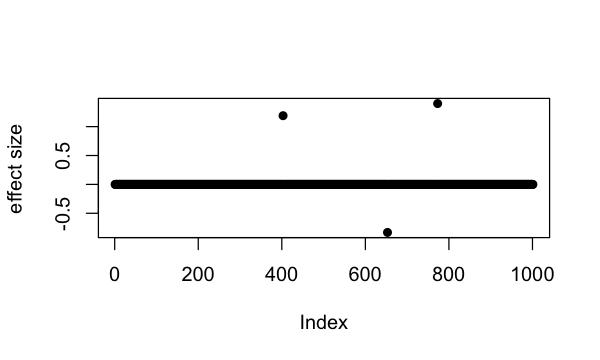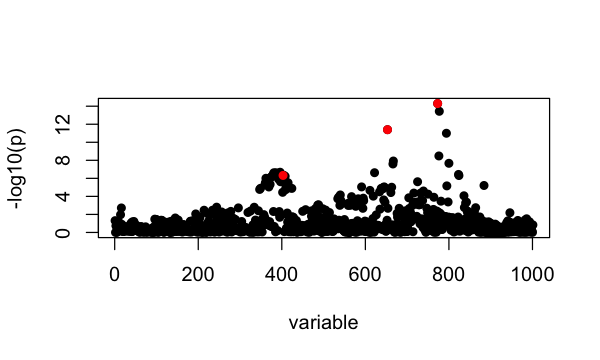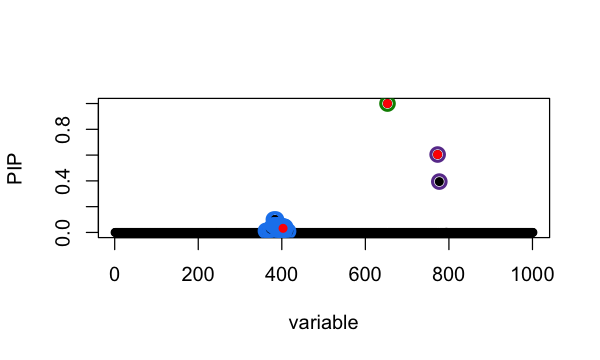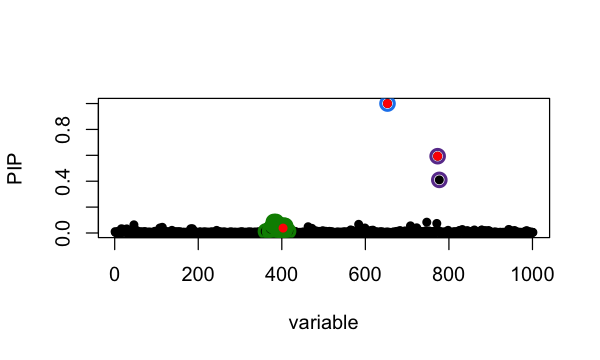# Fine-mapping example

#### 2023-02-17

This vignette demonstrates susieR in the context of genetic fine-mapping. We use simulated data of expression level of a gene ($$y$$) in $$N \approx 600$$ individuals. We want to identify with the genotype matrix $$X_{N\times P}$$ ($$P=1001$$) the genetic variables that causes changes in expression level.

The simulated data set is simulated to have exactly 3 non-zero effects.

library(susieR)
set.seed(1)

## The data-set

data(N3finemapping)
attach(N3finemapping)
# The following objects are masked from N3finemapping (pos = 3):
#
#     allele_freq, chrom, pos, residual_variance, true_coef, V, X, Y

The loaded dataset contains regression data $$X$$ and $$y$$, along with some other relevant properties in the context of genetic studies. It also contains the “true” regression coefficent the data is simulated from.

Notice that we’ve simulated 2 sets of $$Y$$ as 2 simulation replicates. Here we’ll focus on the first data-set.

dim(Y)
#  574   2

Here are the 3 “true” signals in the first data-set:

b <- true_coef[,1]
plot(b, pch=16, ylab='effect size')which(b != 0)
#  403 653 773

So the underlying causal variables are 403, 653 and 773.

## Simple regression summary statistics

univariate_regression function can be used to compute summary statistics by fitting univariate simple regression variable by variable. The results are $$\hat{\beta}$$ and $$SE(\hat{\beta})$$ from which z-scores can be derived. Again we focus only on results from the first data-set:

sumstats <- univariate_regression(X, Y[,1])
z_scores <- sumstats$betahat / sumstats$sebetahat
susie_plot(z_scores, y = "z", b=b)## Fine-mapping with susieR

For starters, we assume there are at most 10 causal variables, i.e., set L = 10, although SuSiE is robust to the choice of L.

The susieR function call is:

fitted <- susie(X, Y[,1],
L = 10,
verbose = TRUE)
#  "objective:-1380.57545244487"
#  "objective:-1377.4866091747"
#  "objective:-1375.85777210115"
#  "objective:-1375.80892303931"
#  "objective:-1370.33949333171"
#  "objective:-1370.19677276994"
#  "objective:-1370.10919739202"
#  "objective:-1370.10918017469"
#  "objective:-1370.10901872278"

### Credible sets

By default, we output 95% credible set:

print(fitted$sets) #$cs
# $cs$L2
#  653
#
# $cs$L1
#  773 777
#
# $cs$L3
#   362 365 372 373 374 379 381 383 384 386 387 388 389 391 392 396 397 398 399
#  400 401 403 404 405 407 408 415
#
#
# $purity # min.abs.corr mean.abs.corr median.abs.corr # L2 1.0000000 1.0000000 1.0000000 # L1 0.9815726 0.9815726 0.9815726 # L3 0.8686309 0.9640176 0.9720711 # #$cs_index
#  2 1 3
#
# $coverage #  0.9998236 0.9988858 0.9539811 # #$requested_coverage
#  0.95

The 3 causal signals have been captured by the 3 CS reported here. The 3rd CS contains many variables, including the true causal variable 403. The minimum absolute correlation is 0.86.

If we use the default 90% coverage for credible sets, we still capture the 3 signals, but “purity” of the 3rd CS is now 0.91 and size of the CS is also a bit smaller.

sets <- susie_get_cs(fitted,
X = X,
coverage = 0.9,
min_abs_corr = 0.1)
print(sets)
# $cs #$cs$L2 #  653 # #$cs$L1 #  773 777 # #$cs$L3 #  373 374 379 381 383 384 386 387 388 389 391 392 396 398 399 400 401 403 404 #  405 407 408 # # #$purity
#    min.abs.corr mean.abs.corr median.abs.corr
# L2    1.0000000     1.0000000       1.0000000
# L1    0.9815726     0.9815726       0.9815726
# L3    0.9119572     0.9726283       0.9765888
#
# $cs_index #  2 1 3 # #$coverage
#  0.9998236 0.9988858 0.9119917
#
# $requested_coverage #  0.9 ### Posterior inclusion probabilities Previously we’ve determined that summing over 3 single effect regression models is approperate for our application. Here we summarize the variable selection results by posterior inclusion probability (PIP): susie_plot(fitted, y="PIP", b=b)The true causal variables are colored red. The 95% CS identified are circled in different colors. Of interest is the cluster around position 400. The true signal is 403 but apparently it does not have the highest PIP. To compare ranking of PIP and original z-score in that CS: i <- fitted$sets$cs[] z3 <- cbind(i,z_scores[i],fitted$pip[i])
colnames(z3) <- c('position', 'z-score', 'PIP')
z3[order(z3[,2], decreasing = TRUE),]
#       position  z-score         PIP
#  [1,]      396 5.189811 0.056704331
#  [2,]      381 5.164794 0.100360243
#  [3,]      386 5.164794 0.100360243
#  [4,]      379 5.077563 0.054179507
#  [5,]      391 5.068388 0.055952118
#  [6,]      383 5.057053 0.052896918
#  [7,]      384 5.057053 0.052896918
#  [8,]      389 5.052519 0.042161265
#  [9,]      405 5.039617 0.045761975
# [10,]      403 5.035949 0.031992848
# [11,]      387 5.013526 0.041041505
# [12,]      388 4.997955 0.039650079
# [13,]      408 4.994865 0.041551961
# [14,]      404 4.954407 0.032013339
# [15,]      374 4.948060 0.030571484
# [16,]      373 4.934410 0.023577221
# [17,]      362 4.894243 0.012145481
# [18,]      399 4.860780 0.026454056
# [19,]      392 4.856384 0.019741011
# [20,]      407 4.849285 0.014699313
# [21,]      400 4.827361 0.021659443
# [22,]      365 4.782770 0.006263425
# [23,]      398 4.751205 0.012907848
# [24,]      401 4.723184 0.014858460
# [25,]      397 4.716886 0.008690915
# [26,]      415 4.663208 0.009003129
# [27,]      372 4.581560 0.005886458

### Choice of priors

Notice that by default SuSiE estimates prior effect size from data. For fine-mapping applications, however, we sometimes have knowledge of SuSiE prior effect size since it is parameterized as percentage of variance explained (PVE) by a non-zero effect, which, in the context of fine-mapping, is related to per-SNP heritability. It is possible to use scaled_prior_variance to specify this PVE and explicitly set estimate_prior_variance=FALSE to fix the prior effect to given value.

In this data-set, SuSiE is robust to choice of priors. Here we set PVE to 0.2, and compare with previous results:

fitted = susie(X, Y[,1],
L = 10,
estimate_residual_variance = TRUE,
estimate_prior_variance = FALSE,
scaled_prior_variance = 0.2)
susie_plot(fitted, y='PIP', b=b)which largely remains unchanged.

### A note on covariate adjustment

To include covariate Z in SuSiE, one approach is to regress it out from both y and X, and then run SuSiE on the residuals. The code below illustrates the procedure:

remove.covariate.effects <- function (X, Z, y) {
# include the intercept term
if (any(Z[,1]!=1)) Z = cbind(1, Z)
A   <- forceSymmetric(crossprod(Z))
SZy <- as.vector(solve(A,c(y %*% Z)))
SZX <- as.matrix(solve(A,t(Z) %*% X))
y <- y - c(Z %*% SZy)
X <- X - Z %*% SZX
return(list(X = X,y = y,SZy = SZy,SZX = SZX))
}

out = remove.covariate.effects(X, Z, Y[,1])
fitted_adjusted = susie(out$X, out$y, L = 10)

Note that the covariates Z should have a column of ones as the first column. If not, the above function remove.covariate.effects will add such a column to Z before regressing it out. Data will be centered as a result. Also the scale of data is changed after regressing out Z. This introduces some subtleties in terms of interpreting the results. For this reason, we provide covariate adjustment procedure as a tip in the documentation and not part of susieR::susie() function. Cautions should be taken when applying this procedure and interpreting the result from it.

## Session information

Here are some details about the computing environment, including the versions of R, and the R packages, used to generate these results.

sessionInfo()
# R version 3.6.2 (2019-12-12)
# Platform: x86_64-apple-darwin15.6.0 (64-bit)
# Running under: macOS Catalina 10.15.7
#
# Matrix products: default
# BLAS:   /Library/Frameworks/R.framework/Versions/3.6/Resources/lib/libRblas.0.dylib
# LAPACK: /Library/Frameworks/R.framework/Versions/3.6/Resources/lib/libRlapack.dylib
#
# locale:
#  en_US.UTF-8/en_US.UTF-8/en_US.UTF-8/C/en_US.UTF-8/en_US.UTF-8
#
# attached base packages:
#  stats     graphics  grDevices utils     datasets  methods   base
#
# other attached packages:
#  susieR_0.12.35
#
# loaded via a namespace (and not attached):
#   tidyselect_1.1.1   xfun_0.29          bslib_0.3.1        purrr_0.3.4
#   lattice_0.20-38    colorspace_1.4-1   vctrs_0.3.8        generics_0.0.2
#   htmltools_0.5.2    yaml_2.2.0         utf8_1.1.4         rlang_1.0.6
#  mixsqp_0.3-46      jquerylib_0.1.4    pillar_1.6.2       glue_1.4.2
#  DBI_1.1.0          RcppZiggurat_0.1.5 matrixStats_0.63.0 lifecycle_1.0.0
#  plyr_1.8.5         stringr_1.4.0      munsell_0.5.0      gtable_0.3.0
#  evaluate_0.14      knitr_1.37         fastmap_1.1.0      parallel_3.6.2
#  irlba_2.3.3        fansi_0.4.0        Rfast_2.0.3        highr_0.8
#  Rcpp_1.0.8         scales_1.1.0       jsonlite_1.7.2     ggplot2_3.3.6
#  digest_0.6.23      stringi_1.4.3      dplyr_1.0.7        grid_3.6.2
#  cli_3.5.0          tools_3.6.2        magrittr_2.0.1     sass_0.4.0
#  tibble_3.1.3       crayon_1.4.1       pkgconfig_2.0.3    ellipsis_0.3.2
#  Matrix_1.2-18      assertthat_0.2.1   rmarkdown_2.11     reshape_0.8.8
#  R6_2.4.1           compiler_3.6.2